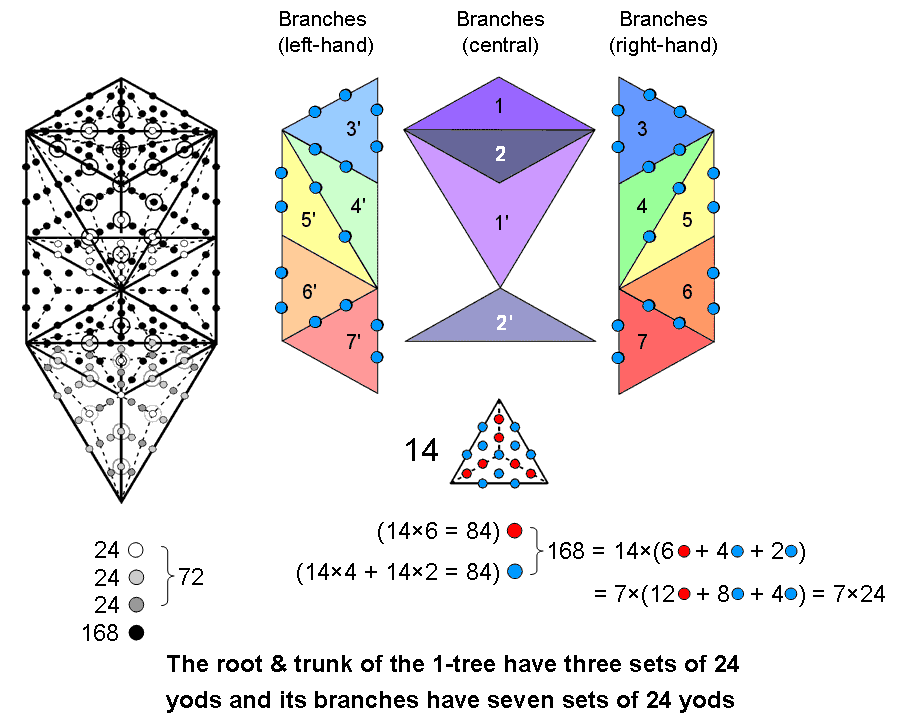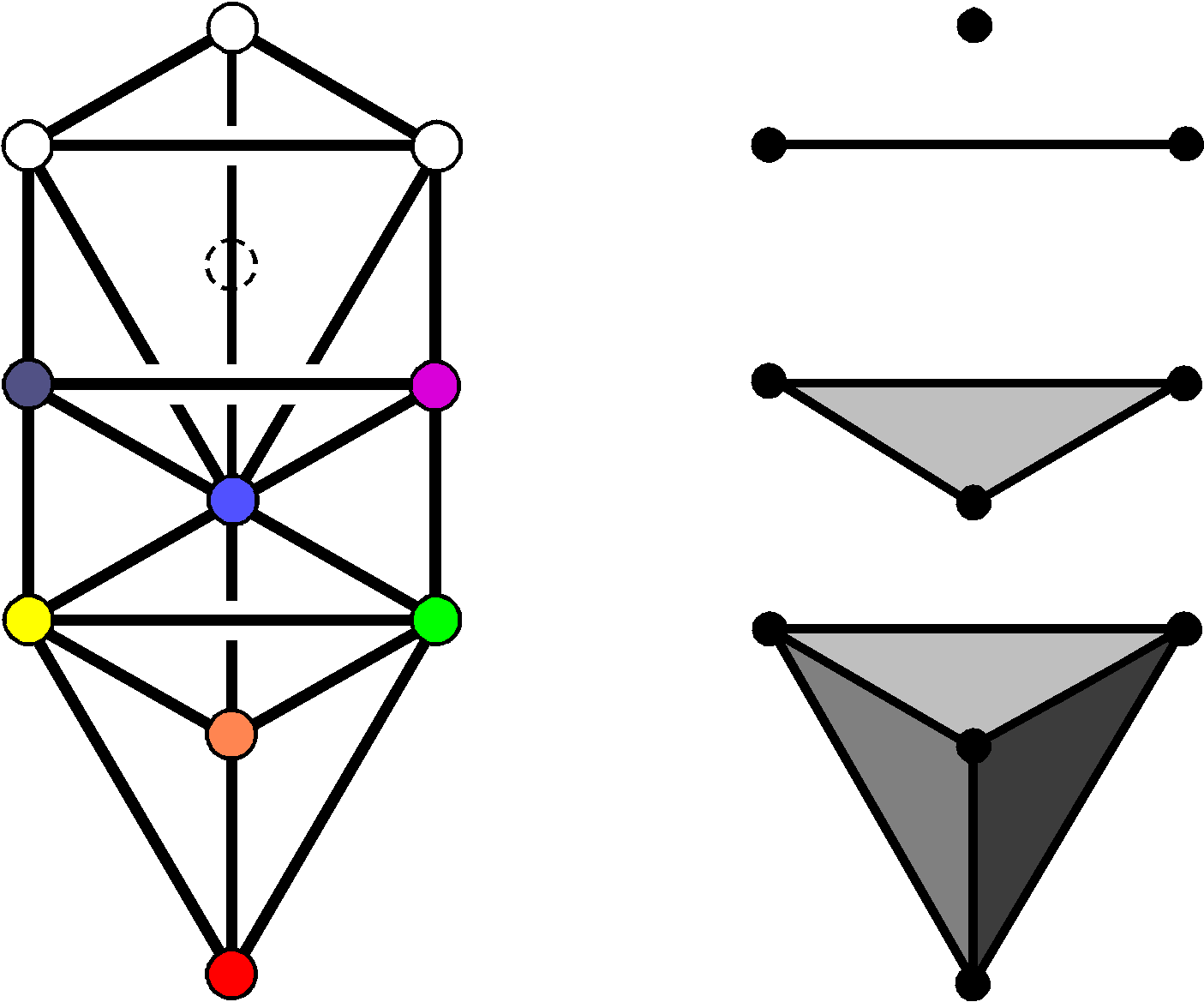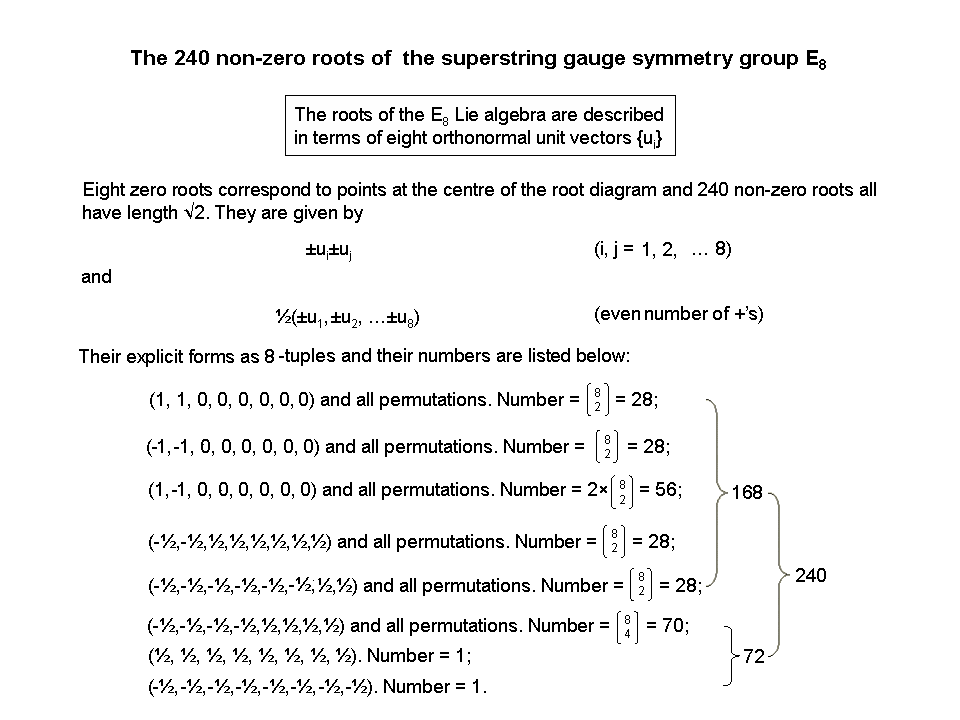<< Previous    1...   35  36    38  39  ...56    Next >>

#37 The 10 sets of 24 yods in the 1-tree correspond to the 10 whorls of the
UPA/superstring along each of which 24 E8 gauge charges are spreadWhen its 19 triangles are turned into Type A triangles, i.e., when each triangle is divided into its three sectors which are then changed into tetractyses, the 1-tree contains 251 yods. Their 11 corners are “Sephirothic levels” (SLs), as Daath is Yesod of the next higher Tree. Hence, the transformation generates (251−11=240) yods that belong to (19×3=57) tetractyses. The 19 triangles have 25 sides. Inside them are (19×3=57) sides of tetractyses. (251−57=194) yods line the (25+57=82) sides of these tetractyses, where 194 is the number value of Tzadekh, the Mundane Chakra of Chesed. The 194 yods comprise (2×82=164) hexagonal yods and (11+19=30) corners. The 1-tree contains (251−30=221) hexagonal yods.

It is explained here that the 240 new yods needed to construct the 1-tree from tetractyses symbolize the 240 roots of the rank-8 exceptional Lie group E8. It will now be shown that these yods form 10 sets of 24 yods, the physical meaning of which will be given later.Tree of Life                    Trunk

The trunk of the Tree of Life consists of the mathematical sequence of the point (Kether), straight line (Chokmah-Binah Path), triangle (corners at Chesed, Geburah & Tiphareth) & tetrahedron (vertices at Netzach, Hod, Yesod & Malkuth). It expresses the emergence of 3-dimensional form from the dimensionless point. The ten points in space needed to achieve this represent the integers 1, 2, 3 & 4, which are also symbolized by the four rows of the Pythagorean tetractys. The trunk is composed of 26 geometrical elements (ten points, ten lines, five triangles & one tetrahedron), where 26 is the number value of YAHWEH, the Godname of Chokmah. When these triangles are Type A triangles, the trunk has 15 triangular sectors with 15 corners & 25 sides, i.e., 55 geometrical elements, where 55 is both the tenth triangular number:

55 = 1 + 2 + 3 + 4 + 5 + 6 + 7 + 8 + 9 + 10

and the tenth Fibonacci number. This demonstrates how the Decad (10) arithmetically determines the geometrical composition of the trunk of the Tree of Life that represents this number. The table below lists the numbers of yods in the trunk other than those coinciding with Sephiroth:

Non-Sephirothic yod population of the trunk of the Tree of Life

 Trunk Corners Hexagonal yods at centres Hexagonal yods on sides Total Point 0 0 0 0 Line 0 0 2 2 Triangle 1 3 12 4 + 12 Tetrahedron 4 12 12 + 24 = 36 4 + (12+12=24) + 24 Total 5 15 50 (2+4+4=10) + 12 + 24 + 24 = 70

ADONAI, the Godname of Malkuth with number value 65, prescribes the trunk because its 15 tetractyses have (15+50=65) hexagonal yods. ELOHIM, the Godname of Binah with number value 50, shapes the trunk because 50 hexagonal yods line its 25 sides. What we will call the 'branches' of the 1-tree are all those parts of the 1-tree other than its trunk. They have (240−70=170) yods. As the 240 yods comprise 19 corners of 57 tetractyses & 221 hexagonal yods, the branches contain (19−5=14) corners & (221−65=156) hexagonal yods in (57−15=42) tetractyses, i.e., (14+156=170) yods. Therefore, the distinction between the trunk & branches of the 1-tree generates the division: 240 = 70 + 170, where 70 = 5 corners + 65 hexagonal yods and 170 = 14 corners + 156 hexagonal yods. The 240 yods include (19+57=76) corners & centres of tetractyses, where 76 is the number value of YAHWEH ELOHIM, the Godname of Tiphareth. The complete 1-tree has (11+19=30) corners and 57 centres of tetractyses, i.e., 87 corners & centres, where 87 is the number value of Levanah, the Mundane Chakra of Yesod.

According to the table, the number of yods in the trunk is:

70 = 10 + 12 + 24 + 24,

where:

• '10' denotes the two hexagonal yods in the 'line' (Path joining Chokmah and Binah), the centre of the 'triangle', the three hexagonal yods at the centres of its sectors and the four centres of the faces of the tetrahedron;
• '12' denotes the 12 hexagonal yods on the sides of the three tetractyses making up the 'triangle';
• '24' denotes the 24 hexagonal yods either at the centres of the tetractyses in the tetrahedron or on its edges;
• '24' denotes the 24 hexagonal yods on sides of tetractyses in the tetrahedron other than its edges.

Suppose that we add the two white hexagonal yods on the Path joining Daath and Tiphareth. The projection of this line onto the plane of the (7+7) enfolded polygons making up the inner Tree of Life is the side that they all share — what we have called the "root edge," the generative source of the 14 polygons. This Path is its counterpart in the outer Tree of Life. For this reason, it will be called the "root" of the latter. The trunk and root constitute a set of 72 yods:

72 = 2 + 70 = (2+10) + 12 + 24 + 24 = (12+12) + 24 + 24 = 24 + 24 + 24.

We see that they consist of three sets of 24 yods. They are shown in the diagram above as coloured white, light grey & dark grey. This leaves (170−2=168) yods in the branches per se made up of 14 corners and 154 hexagonal yods in 14 Type A triangles with two hexagonal yods on each of their 14 sides (there are actually 15 sides outside the trunk but one is the line connecting Daath & Tiphareth which we are calling the 'root' of the outer Tree of Life. There are nine hexagonal yods inside each Type A triangle, so that the 168 yods comprise 14 corners, 14 sets of nine hexagonal yods and 14 pairs of hexagonal yods, i.e., 14 sets of 12 yods, or seven sets of 24 yods. Each set of 24 yods comprises two centres of triangles of the 1-tree, four hexagonal yods on two Paths & 18 hexagonal yods inside two triangles. The latter consist of six hexagonal yods at centres of six tetractyses & 12 hexagonal yods on six sides of them, so that the 24 yods comprise two corners of tetractyses, six hexagonal yods at their centres and (4+12=16) hexagonal yods on their sides. This 2:6:16 pattern is identical to what is found for the 24 transverse dimensions of 26-dimensional bosonic strings, for they comprise two large-scale dimensions, the six dimensions of the compactified space of superstrings and 16 higher dimensions. The following correspondences exist:

1. 2 corners of tetractyses ↔ 2 large-scale, transverse dimensions;
2. 6 hexagonal yods at centres of tetractyses ↔ 6 compactified, superstring dimensions;
3. 16 hexagonal yods on sides of tetractyses ↔ 16 compactified, bosonic string dimensions.

This is not coincidental, because the 240 yods symbolize the 240 roots of E8, whose associated gauge charges are spread along the ten whorls of the UPA/E8×E8 heterotic superstring, 24 to a whorl, and each gauge charge is the result of an oscillation of a whorl along each of the 24 transverse dimensions of space. Nevertheless, the analogy between the hidden yod composition of the 1-tree and the pattern of dimensions of space predicted by the theories of bosonic strings and superstrings is remarkable.Notice that the 72 white yods, which symbolize the 72 roots of E6, the rank-6, exceptional subgroup of E8, have been compounded from a set of 70 yods making up the trunk of the 1-tree and the two hexagonal yods on the Daath-Tiphareth Path. The 72 roots also consist of a set of 70 roots and two roots represented by the 8-tuples (½, ½, ½, ½, ½, ½, ½, ½) and (-½, -½, -½, -½, -½, -½, -½, -½) (see diagram). Each is the mirror reflection of the other in the 8-dimensional vector space. The two hexagonal yods in the root project onto the two hexagonal yods in the root edge of the inner Tree of Life, one of which is the mirror image of the other, the two sets of seven polygons separated by the root edge being mirror images of each other. So the group-theoretical composition of the 72 roots is paralleled by the yod composition of the trunk and the root of the 1-tree.

So we find that the 240 yods needed to construct the 1-tree from Type A triangles form ten sets of 24 yods, which divide further into three sets of 24 (root & trunk) and seven sets of 24 (branches). This 3:7 division is the Tree of Life counterpart of the three major whorls and seven minor whorls of the UPA/subquark state of the E8×E8 heterotic superstring. The three major whorls with 72 E8 gauge charges are the manifestation of the root and trunk of the 1-tree with 72 yods; the seven minor whorls with 168 gauge charges are the manifestation of its branches with 168 yods. The factorization: 168 = 7×24 is made possible by the facts that: 1. the branches of the 1-tree contain 14 triangles, and 2. each side of the 1-tree is the mirror image of the other, so that it can be regarded as two sets of seven triangles. Five triangles (coloured dark or light shades of red, orange, yellow, green & blue) are on each side of the central Pillar of Equilibrium, which is straddled by four triangles coloured light & dark shades of indigo and violet. The dark shades of these two colours can be associated with one side of the 1-tree and their light shades can be associated with its other side. Therefore, the factor of seven in the factorization arises simply from the fact that seven triangles can be associated with the left-hand side of the 1-tree and seven triangles can be associated with its right-hand side. There are two hexagonal yods on a side shared by two triangles. One hexagonal yod is associated with one triangle and the second one should be regarded as associated with the other triangle. This allows 12 yods to be associated with each triangle. Each set of 24 yods consists of 12 yods associated with a triangle in each side of the 1-tree, so that the 168 yods divide into two sets of 84 yods associated with each half of it. This 84:84 division is characteristic of sacred geometries (see The holistic pattern). It manifests in the subquark as the outer and inner halves of each helical whorl of the UPA, each having 840 circular turns that make 2½ revolutions around its axis of spin, that is, 168 turns make a half-revolution and 84 turns make a quarter-revolution. The list of the 240 roots of E8 shown opposite indicates that the 168 roots of E8 that are not roots of E6 consist of three sets of 28 roots (i.e., 84 roots), a set of 56 roots and another set of 28 (i.e., 84 roots). So these 168 roots divide naturally into two sets of 84 roots. Their counterparts in the branches of the 1-tree are the 84 yods that belong to the seven triangles associated with its left-hand or right-hand half. Alternatively, as there are six red hexagonal yods on sides of tetractyses inside each of the 14 triangles making up the branches, the latter have 84 red hexagonal yods, leaving 84 blue yods (see diagram). Which is the correct assignment? The correspondence between the former 84:84 division of the 168 yods, which is one based up splitting the outer Tree of Life into its two halves related by a modified mirror symmetry, and the fact that the outer and inner halves of a whorl comprise 840 (=84×10) circular turns, seems to be more apt than the latter, which distinguishes only yods and makes no distinction about the left- or right-hand side of the Tree of Life.

Inspection of the 1-tree reveals that four corners of tetractyses, four hexagonal yods at their centres & eight hexagonal yods on their sides (i.e., 16 yods) are aligned with the central Pillar of Equilibrium, which is straddled by four of the 14 triangles of the branches. Hence, (16816=152) yods belong to the branches in both halves of the 1-tree. 76 yods in the branches outside the Pillar of Equilibrium belong to each half, where 76 is the number value of YAHWEH ELOHIM.

 << Previous    1...   35  36    38  39  ...56    Next >>

Home